# Electronics and Communication Engineering - Exam Questions Papers

11.

Find RAB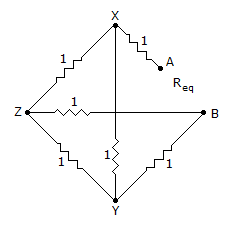A. 1 Ω B.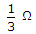C.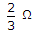D. 2 Ω

Explanation:

By solving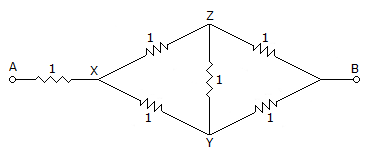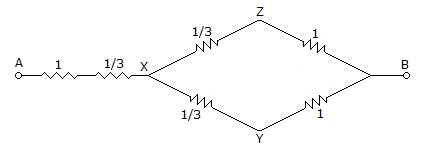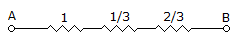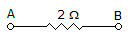12.

For the network shown below, determine fHi and fHO, if, r0 = ∞, Cbe = 36 Pf, Cbc = 4 Pf, Cce = 1 Pf, Cwo = 8 Pf, Cwi = 6 Pf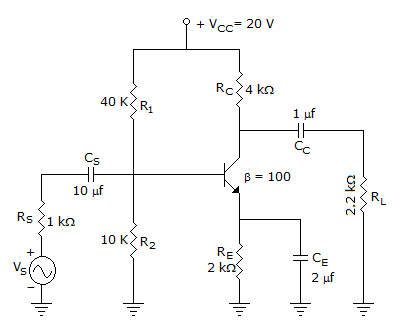where Cwo = wiring capacitance at output side, Cwi = wiring capacitance at input side

 A. 738.24 KHz, 8.6 MHz B. 781.2 KHz, 10.6 MHz C. 838.32 KHz, 9.2 MHz D. 938.24 KHz, 8.2 MHz

Explanation:

Equivalent is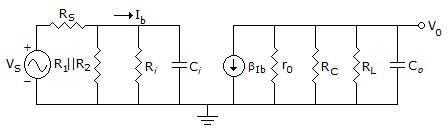RTi = RS || R1 || R2 || Ri = 0.531 KΩ

Ci = Cwi + Cbe + (1 + AV)Cbc

= 6pf + 36pf + (1 - c - 90) 4 pf = 460 pf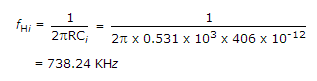RT2 = RC || RL = 4 K || 2.2 K = 1.419 KW

C0 = Cwo + Cce + CMO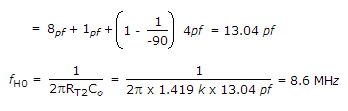13.

Complex pole in z-plane is as shown below. This is the pole diagram for __________ .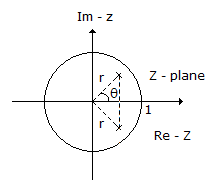A. Decaying sinusoidal sequence B. Growing sinusoidal sequence C. Decaying non sinusoidal sequence D. Growing non sinusoidal sequence

Explanation:

Decaying sinusoidal sequence.

14.

Four messages band limited to W, W, 2W and 3W respectively are to be multiplex using Time Division Multiplexing (TDM). The minimum bandwidth required for transmission of this TDM signal is

 A. W B. 3W C. 6W D. 7W

Explanation:

In TDM minimum bandwidth is twice the maximum frequency present. Thus, 6W is the answer.

15.

Which of the following terms are equivalent?

1. A ⊕ B ⊕ C
2. A ⊕ B ⊙ C
3. A ⊙ B ⊕ C
4. A ⊙ B ⊙ C

 A. 1 and 4; 2 and 3 B. 1 and 3 C. 2 and 3 D. 1, 2 and 4

Explanation:

A ⊕ B ⊕ C = A (B ⊕ C) + A(B ⊕ C)

= A(B ⊙ C) + A (B ⊙ C) = A ⊙ B ⊙ C

A ⊕ B ⊙ C = A (B ⊙ C) + A(B ⊙ C)

= A(B ⊕ C) + A(B ⊕ C)

= A ⊙ B ⊙ C.

#### Current Affairs 2022

Interview Questions and Answers